# Costa-Hoffman-Meeks Surface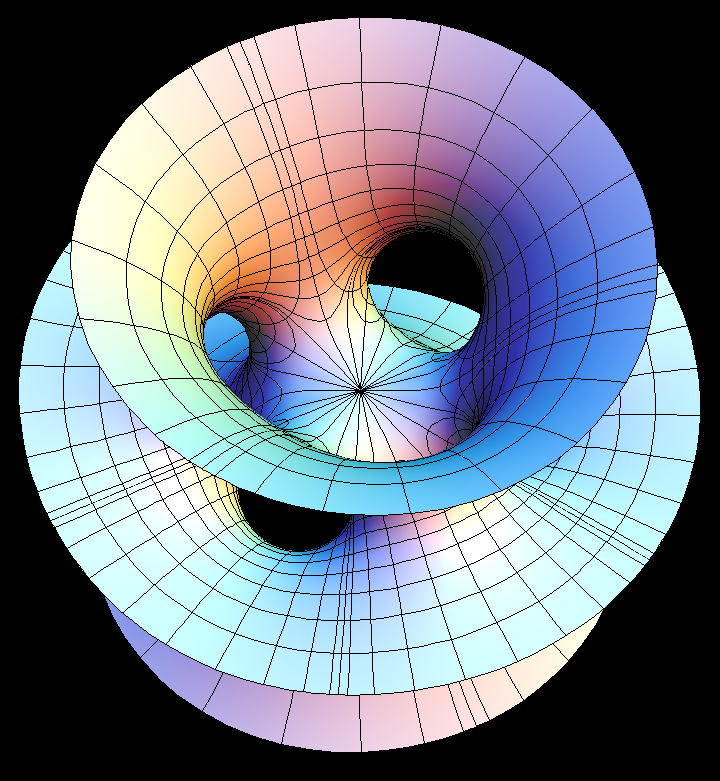Costa-Hoffman-Meeks Surface, viewed from different angles

Costa-Hoffman-Meeks Surface is generailization of the Costa Surface to higher dihedral symmetry.

Costa-Hoffman-Meeks Surface with dihedral symmetry n has genus n-1.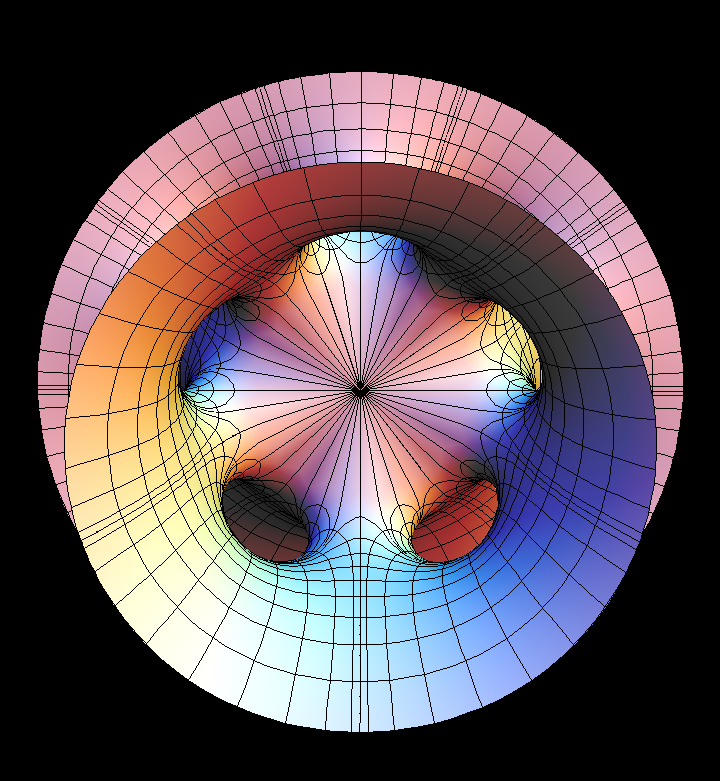Costa-Hoffman-Meeks with 5-fold symmetry, viewed from different angles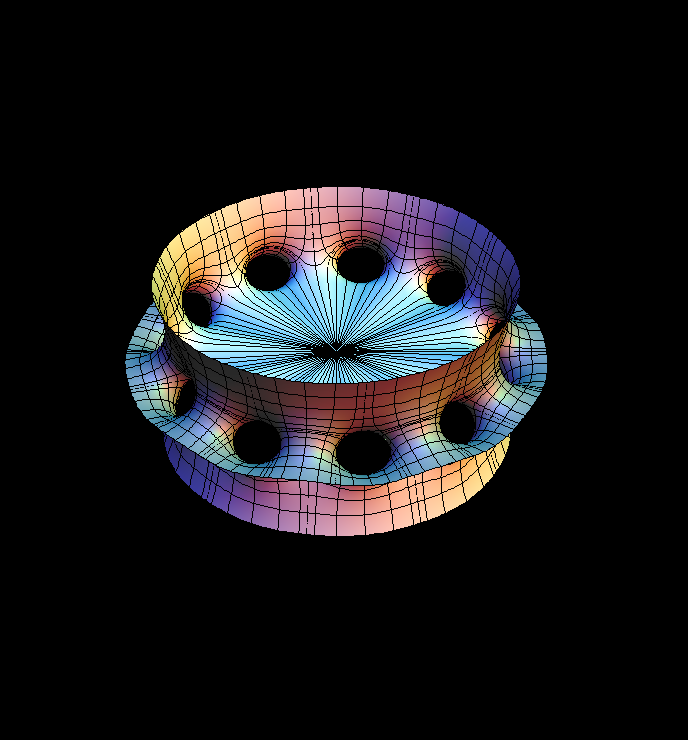Costa-Hoffman-Meeks with 10-fold symmetry, with increasing plot range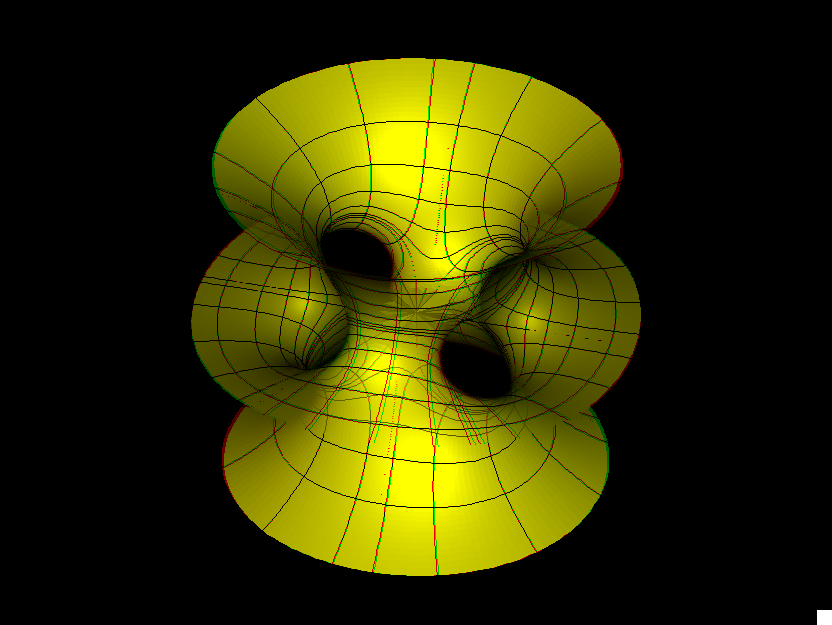costa h m st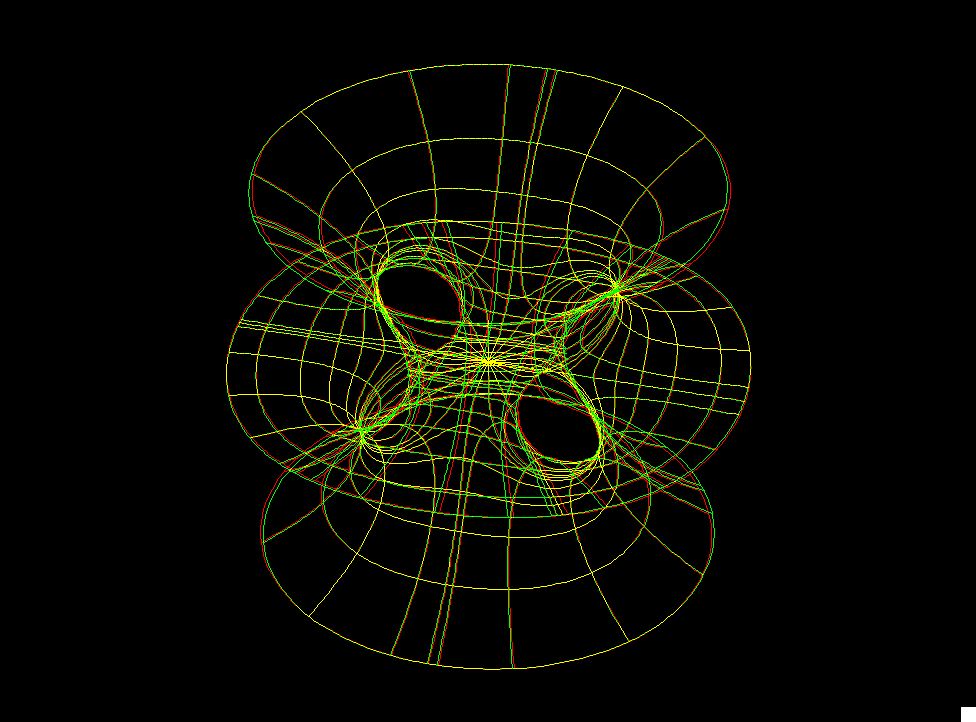costa h m sw

The original Costa Surface was responsible for the rekindling of interest in minimal surfaces in 1982. It is a minimal EMBEDDING of the 3-punctured square Torus. Its planar symmetry lines cut this surface into four conformal squares and the two straight lines through the saddle are the diagonals of these squares. Because of the emphasis on the symmetries, our formulas are taken from [K2.]

The Costa-Hoffman-Meeks surfaces are generalizations of the Costa Surface; their genus grows as the dihedral symmetry (controlled by dd) is increased. The underlying Riemann surfaces are tesselated by hyperbolic squares with angles pi/k, (k = 2,3, ...).

The Gauss map of such a surface is determined by its qualitative properties only up to a multiplicative factor cc which we suggest for the morphing (as in the Chen Gackstatter case). It closes the period (at cc0) with an intermediate value argument.

• [Hoffman, Karcher] Complete embedded minimal surfaces of finite total curvature. Encyclopaedia of Mathematical Sciences, vol. 90. Geometry V (Ed. R. Osserman), pp. 5-93
• [K2] Construction of Minimal Surfaces, H Karcher , in “Surveys in Geometry”, Univ. of Tokyo, 1989, and Lecture Notes No. 12, SFB 256, Bonn, 1989, pp. 1 — 96.

For a discussion of techniques for creating minimal surfaces with various qualitative features by appropriate choices of Weierstrass data, see either [KWH], or pages 192--217 of [DHKW].

• [KWH] H. Karcher, F. Wei, and D. Hoffman, The genus one helicoid, and the minimal surfaces that led to its discovery, in “Global Analysis in Modern Mathematics, A Symposium in Honor of Richard Palais' Sixtieth Birthday”, K. Uhlenbeck Editor, Publish or Perish Press, 1993
• [DHKW] U. Dierkes, S. Hildebrand, A. Kuster, and O. Wohlrab, Minimal Surfaces I, Grundlehren der math. Wiss. v. 295 Springer-Verlag, 1991

Get red/blue stereo glasses from amazon

Costa-Hoffman-Meeks.pdf